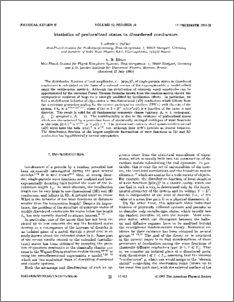# Statistics of prelocalized states in disordered conductors

Falko, Vladimir and Efetov, K. B. (1995) Statistics of prelocalized states in disordered conductors. Physical Review B, 52 (24). pp. 17413-17429. ISSN 0163-1829Preview
PDF (PhysRevB.52.17413) - Published Version
Download (690Kb) | Preview

## Abstract

The distribution function of local amplitudes, t = \psi(r(0))\(2), of single-particle states in disordered conductors is calculated on the basis of a reduced version of the supersymmetric sigma model solved using the saddle-point method. Although the distribution of relatively small amplitudes can be approximated by the universal Porter-Thomas formulas known from the random-matrix theory, the asymptotical statistics of large t's is strongly modified by localization effects. In particular, we find a multifractal behavior of eigenstates in two-dimensional (2D) conductors which follows from the noninteger power-law scaling for the inverse participation numbers (IPN's) with the size of the system, Vt(n) proportional to L(-(n-1)d*(n)), where d*(n) = 2 - beta(-1)n/(4 pi nu D) is a function of the index n and disorder. The result is valid for all fundamental symmetry classes (unitary, beta(u) = 1; orthogonal, beta(o) = 1/2; symplectic, beta(s) = 2). The multifractality is due to the existence of prelocalized states which are characterized by a power-law form of statistically averaged envelopes of wave functions at the tails, \psi(t)(r)\(2) proportional to r(-2 mu), mu = mu(t) < 1. The prelocalized states in short quasi-one-dimensional (1D) wires have the tails \psi(x)\(2) proportional to x(-2), too, although their IPN's indicate no fractal behavior. The distribution function of the largest-amplitude fluctuations of wave functions in 2D and 3D conductors has logarithmically normal asymptotics.

Item Type: Journal Article Physical Review B © 1995 The American Physical Society ?? qc ?? Faculty of Science and Technology > Physics 57077 ep_importer_pure 05 Sep 2012 17:39 Yes Published 18 Dec 2018 00:19 http://eprints.lancs.ac.uk/id/eprint/57077

### Actions (login required)

 View Item